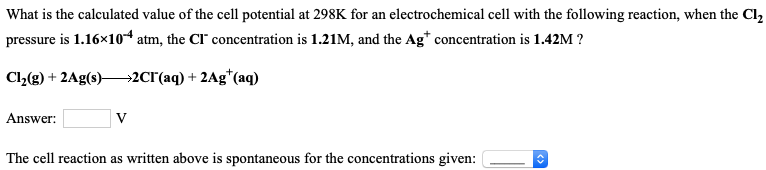# What is the calculated value of the cell potential at 298 K for an electrochemical cell with the following reaction, when the Cl2 pressure is 1.6x10^-4 atm, the Cl^- concentrations is 1.21 M, and the Ag^+ concentration is 1.42M? Cl2(g) + 2Ag(s) → 2Cl^-(aq) + 2Ag^+(aq) The cell reaction as written above is spontaneous for the concentrations given: